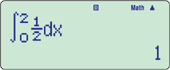# Casio fx-570ES PLUS Calculator

2,175.00

### Casio fx-570ES PLUS Calculator

SKU: FX-570ES Category: Tags: , , ,

## Features

### New functions

• New equation mode
• Random integers

### Standard functions

• Fraction calculations
• Combination and permutation
• Statistics (List-based STAT data editor, standard deviation, regression analysis)
• 9 variables
• Table function
• Comes with new slide-on hard case

### fx-82ES PLUS/85ES PLUS/350ES PLUS functions, in addition to:

• Equation calculations
• Integration/differential calculations
• Matrix calculations
• Vector calculations
• Complex number calculations
• CALC function
• SOLVE function
• Base-n calculation

#### Integration#### Complex number calculations#### Differential#### Vector#### Matrix operations## Specifications

•GCSE/AS/A LevelSuitable for Cambridge International Examinations GCSE, AS & A Level.

•AAA × 1 (R03)

•Number of Functions : 417

•Natural textbook displayDisplay expression same as textbook.

•10 + 2 digits10-digit mantissa + 2-digit exponential display.

•Dot matrix displayHigh-resolution screen provides beautiful looking graphs every time.

•Multi-replayQuick and easy recall of previously executed formulas for editing and re-execution.

•Plastic keysDesigned and engineered for easy operation.

•List based STAT-data editorViewing and editing of input data in list format, showing data groups (x-data, y-data, frequency) and surrounding data.